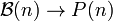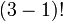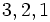Combinatorics of symmetric group:S3

View combinatorics of particular groups | View other specific information about symmetric group:S3

This page discusses some of the combinatorics associated with symmetric group:S3, that relies specifically on viewing it as a symmetric group on a finite set. For more on the element structure from a group-theoretic perspective, see element structure of symmetric group:S3.

Partitions, subset partitions, and cycle decompositions

Denote by$\mathcal{B}(n)$ the set of all unordered set partitions of$\{ 1,2,\dots,n\}$ into subsets and by$P(n)$ the set of unordered integer partitions of$n$. There are natural combinatorial maps:$S_n \to \mathcal{B}(n) \to P(n)$

where the first map sends a permutation to the subset partition induced by its cycle decomposition, which is equivalently the decomposition into orbits for the action of the cyclic subgroup generated by that permutation on$\{ 1,2,\dots,n\}$. The second map sends a subset partition to the partition of$n$ given by the sizes of the parts. The composite of the two maps is termed the cycle type, and classifies conjugacy classes in$S_n$, because cycle type determines conjugacy class.

Further, if we define actions as follows:

•$S_n$ acts on itself by conjugation
•$S_n$ acts on$\mathcal{B}(n)$ by moving around the elements and hence changing the subsets
•$S_n$ acts on$P(n)$ trivially

then the maps above are$S_n$-equivariant, i.e., they commute with the$S_n$-action. Moreover, the action on$\mathcal{B}(n)$ is transitive on each fiber above$P(n)$ and the action on$S_n$ is transitive on each fiber above the composite map to$P(n)$. In particular, for two elements of$\mathcal{B}(n)$ that map to the same element of$P(n)$, the fibers above them in$S_n$ have the same size.

There are formulas for calculating the sizes of the fibers at each level.

In our case$n = 3$:

Partition Partition in grouped form Formula calculating size of fiber for$\mathcal{B}(n) \to P(n)$ Size of fiber for$\mathcal{B}(n) \to P(n)$ Formula calculating size of fiber for$S_n \to \mathcal{B}(n)$ Size of fiber for$S_n \to \mathcal{B}(n)$ Formula calculating size of fiber for$S_n \to P(n)$, which is precisely the conjugacy class size for that cycle type (product of previous two formulas) Size of fiber for$S_n \to P(n)$, which is precisely the conjugacy class size for that cycle type (product of previous two sizes)
1 + 1 + 1 1 (3 times)$\frac{3!}{(1!)^33!}$ 1$(1 - 1)!^3$ 1$\frac{3!}{(1)^33!}$ 1
2 + 1 2 (1 time), 1 (1 time)$\frac{3!}{(2!)(1!)}$ 3$(2 - 1)!(1 - 1)!$ 1$\frac{3!}{(2)(1)}$ 3
3 3 (1 time)$\frac{3!}{3!}$ 1$(3 - 1)!$ 2$\frac{3!}{3}$ 2
Total (3 rows -- number of rows equals number of unordered integer partitions of 3) -- -- 5 (equals the size of$\mathcal{B}(n)$, termed the Bell number, at$n = 3$) -- 4 (see Oeis:A107107) -- 6 (equals 3!, the size of the symmetric group)

Young diagrams and tableaux under the Robinson-Schensted correspondence

Summary

Partition Number of Young tableaux for that shape Hook length formula Number of permutations (via Robinson-Schensted correspondence) equals square of number of Young tableaux List of permutations (each permutation written using one-line notation)
1 + 1 + 1 1$\frac{3!}{3 \cdot 2 \cdot 1}$ 1$[1,2,3]$
2 + 1 2$\! \frac{3!}{3 \cdot 1 \cdot 1}$ 4$[1,3,2]$,$[3,1,2]$,$[2,1,3]$,$[2,3,1]$
3 1$\! \frac{3!}{3 \cdot 2 \cdot 1}$ 1$[3,2,1]$

Note that the numbers in the first column are also the degrees of irreducible representations, see linear representation theory of symmetric groups and linear representation theory of symmetric group:S3.

Partition details

Further information: Robinson-Schensted correspondence for symmetric group:S3

Here the partition is the partition for the Young diagram under the Robinson-Schensted correspondence, not the partition for the cycle type of the permutation.

Permutation (one-line notation) Partition (Young diagram) Position tableau Shape tableau$1,2,3$ 1 + 1 + 1$\begin{pmatrix}1 \\ 2 \\ 3 \\\end{pmatrix}$$\begin{pmatrix} 1 \\ 2 \\ 3 \\\end{pmatrix}$$2,1,3$ 2 + 1$\begin{pmatrix}1 & 2 \\ 3 & \\\end{pmatrix}$$\begin{pmatrix}1 & 2 \\ 3 & \\\end{pmatrix}$$1,3,2$ 2 + 1$\begin{pmatrix}1 & 3 \\ 2 & \\\end{pmatrix}$$\begin{pmatrix} 1 & 3 \\ 2 & \\\end{pmatrix}$$2,3,1$ 2 + 1$\begin{pmatrix}1 & 2 \\ 3 & \\\end{pmatrix}$$\begin{pmatrix} 1 & 3 \\ 2 & \\\end{pmatrix}$$3,1,2$ 2 + 1$\begin{pmatrix}1 & 3 \\ 2 & \\\end{pmatrix}$$\begin{pmatrix} 1 & 2 \\ 3 & \\\end{pmatrix}$$3,2,1$ 3$\begin{pmatrix} 1 & 2 & 3 \\\end{pmatrix}$$\begin{pmatrix} 1 & 2 & 3 \\\end{pmatrix}$

Ascent/descent patterns

One-line notation for permutation Ascent/descent pattern (whether each element is greater or smaller than its predecessor; an A denotes ascent, a D denotes decrease) Number of Is
1,2,3 AA 2
2,1,3 DA 1